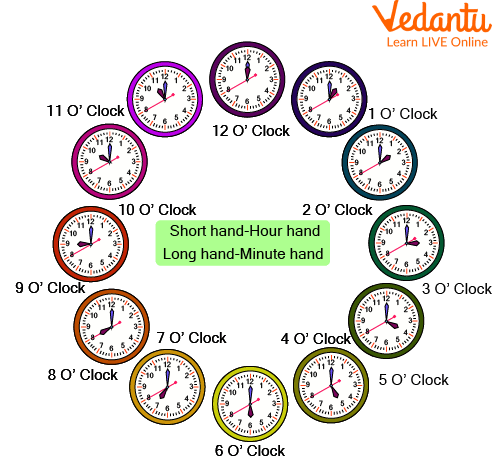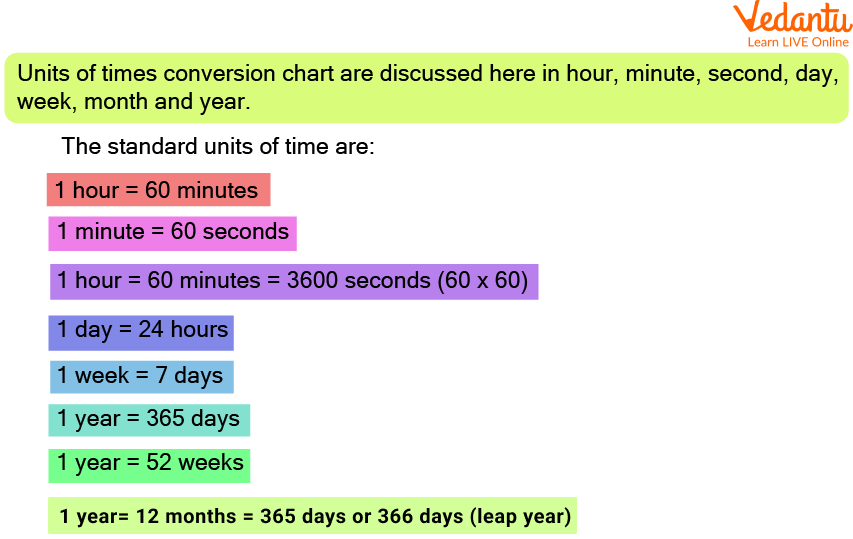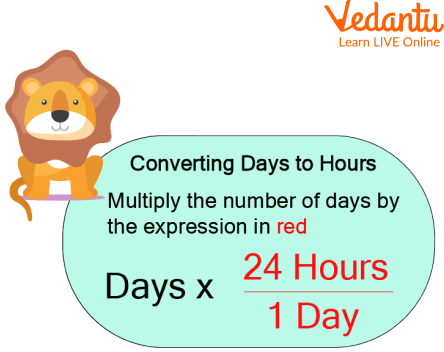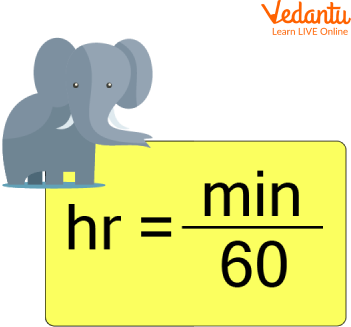Courses
Courses for Kids
Free study material
Offline Centres
More

# How to Convert Hours Into Days?Last updated date: 03rd Dec 2023
Total views: 98.7k
Views today: 1.98k## Introduction to Time Conversion

Time is defined as the duration in which all things happen, or a precise instant that something happens. An example of time is breakfast at eight o'clock in the morning. An example of time is a date at noon next Saturday. Two kinds of clocks are used to see the time. Analog clock and Digital clock.Analog clock has a minute hand,hour hand and sometimes a second hand also. Now, the digital clock on the left side shows the hours from 1 to 12 and the right side shows the minutes from 00 to 59.

Some useful terms A.M.(ante meridian; before midday) It is the time between midnight and noon. P.M.(post meridian: after midday) It is the time between noon and midnight.Time on Clock

## How Many Minutes Are in an Hour?

Seconds: Second is the basic unit of time. There are 60 seconds in 1 minute and 3,600 seconds in an hour.

Minutes: There are 60 minutes in an hour, that is why we use this value in the formula. 1 hr = 60 mins.

Hours: The hour is a period of time equal to $\dfrac{1}{24}$ of a day or 60 minutes. The hour is an SI-accepted unit for time for use with the metric system. Hours can be abbreviated as hr; for example, 1 hour can be written as 1 hr.

## Units for Measuring Time

Some of the basic units of time are second, minute, hour, day, week, month, and year. We estimate time using different units depending on the span of time we are trying to measure.

Minute(min) 1min=60s

Hour (h) 1h=60 min

Day (d) 1d=24h

1 week = 7 days

1 month= 28 or 29 or 30 or 31 days

1 year= 12 months = 365 days or 366 days (leap year)

Below is the units of time chart:Units of time chart

Now we will learn how we can convert days to hours and minutes.

## To convert days into hours, multiply the time by the conversion ratio.

Since one day is equal to 24 hours, you can use this simple formula to convert:

hours = days $\times$ 24

The time in hours is equal to the days multiplied by 24.

For example, here's how to convert 5 days to using the formula above.

5 day = (5 $\times$ 24) = 120 hr

1day = 24hrsFormula of converting days to hours.

Example: 5 days in hours.

5 days = 5 $\times$ 1 day

Since, 1 day there is 24hrs.

Therefore, for 5 days, it will be

= 5 $\times$ 24 hrs

5 days = 120 hrs

## How to Convert Hours Into Days?

To convert an hour measurement to a day measurement, divide the time by the conversion ratio.

1 hr = $\dfrac{1}{24}$ days

Here's how to convert 76 hrs to days using the formula above.

76 hrs = 76 $\times$ 1 hr

$= 76 \times \dfrac{1}{24}$ days

$= \dfrac{76}{24}$ days

= 3 days 4 hrs

## How to Convert Hours to Minutes?

The rule for converting hours to minutes is very simple, just multiply the number of hours by 60 and you will get the time in minutes. For example, to convert 2 hours into minutes, multiply 2 by 60, and you will get 120 minutes.

Example:

Convert 6 hours into minutes.

We know, 1 hour = 60 minutes

Therefore, 6 hours = (6 $\times$ 60) minutes = 360 minutes.

## How to Convert Minutes to Hours?

To convert minutes to hours and minutes,

use long division to divide the minutes by 60, answer quotients are the hours part the answer remainder is the minutes part.Formula to convert minutes to hours.

Total minutes $\div 60=\mathrm{Q}$ hours and $\mathrm{R}$ minutes

Example: To show an example and how it works, let's say we want to convert 190 minutes to hours and minutes.

190 divided by 60 equals 3 with a remainder of 10.

The quotient Q = 3 and the remainder R = 10. So, 190 minutes = 3 hours and 10 minutes.

## Solved Examples

Q 1. Write 1:23 pm in 24-hour format. Write 4:56 in 12-hour format.

Ans: If we add 12 hours i.e. 12.00 to a 12 hour format time only am pm will be the same then the result in 24-hour format.

Therefore, 1:23 + 12 = 13:23 in 24 hour format.

4:56 hours in 12 hour format = 4:56 am

Q 2. Convert 45 minutes into an hour.

Ans: We know,

60 minutes = 1 hour

or, 1 minute = $\dfrac{1}{60}$ hour

Therefore, 45 minutes = $\dfrac{45}{60}$ hour

= $\dfrac{3}{4}$ hour

Q 3. Convert 9 hours 35 minutes into minutes.

Ans: First, we convert 9 hours into minutes and then we add 35 minutes to it.

9 hours 35 minutes = (9 × 60) minutes + 35 minutes (1 hour = 60 minutes)

= 540 minutes + 35 minutes

= 575 minutes

Q 4. Convert 7 days 6 hours into hours.

Ans: 1 day = 24 hours

Therefore, 7 days 6 hour

= (7 x 24)hours+ 6 hours

= 168 hours ie 6 hours = 174 hours

Q 5. Convert 5 min 37 sec into seconds.

Ans: 1 min = 60 sec

Therefore, 5 min = 5 x 60 = 300 sec

5 min 37 sec = 300 sec + 37 sec = 337 seconds

## Practice Questions

Q 1. Convert 180 minutes to hours.

Ans: 3 hours

Q 2. Convert 240 seconds into minutes.

Ans: 4 minutes

Q 3. A can do a piece of work in 12 days. B can do this work in 16 days. A started the work alone. After how many days should B join him, so that the work is finished in 9 days?

Ans: 5 days

Q 4. School starts at 8:00 a.m. and closes at 12:45 a.m. How long does it work?

Ans: 4 hours 45 minutes.

Q 5. What time will it be: 6 hours 35 minutes after 11:10 am?

Ans: 5:45 pm

## Summary

Time can be defined as an ongoing and continuous sequence of events that occur in succession, from past through present, and to the future. Time is used to quantify, measure or compare the duration of events or the intervals between them, and even, sequence events. Time can be represented in two formats: 24-hour format and 12-hour format. In 12-hour format, am stands for ‘ante meridiem’, which is Latin for ‘before midday’. pm stands for ‘post meridiem’, which is Latin for ‘after midday’.Comparison of 12-hour and 24-hour conventions of time.In this chapter we have studied about conversions of days and hours. Hope you all have understood the concept of time,for any queries ask in the comment section.

## FAQs on How to Convert Hours Into Days?

1. What is telling the time?

The ability to read from a clock the time of day or night in units of hours, minutes and seconds.

2. How do we measure time?

We can measure time intervals most accurately with atomic clocks.

3. Who came up with time?

The measurement of time began with the invention of sundials in ancient Egypt some time prior to 1500 B.C. However, the time the Egyptians measured was not the same as the time today's clocks measure. For the Egyptians, and indeed for a further three millennia, the basic unit of time was the period of daylight.